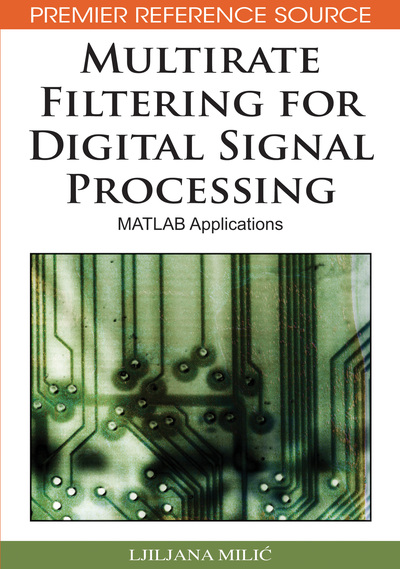# Lth-Band Digital Filters

Ljiljana Milic (Mihajlo Pupin Institute, Serbia)
DOI: 10.4018/978-1-60566-178-0.ch007
Available
\$33.75
List Price: \$37.50
10% Discount:-\$3.75
TOTAL SAVINGS: \$3.75

## Abstract

Digital Lth-band FIR and IIR filters are the special classes of digital filters, which are of particular interest both in single-rate and multirate signal processing. The common characteristic of Lth-band lowpass filters is that the 6 dB (or 3 dB) cutoff angular frequency is located at p/L, and the transition band is approximately symmetric around this frequency. In time domain, the impulse response of an Lth-band digital filter has zero valued samples at the multiples of L samples counted away from the central sample to the right and left directions. Actually, an Lth-band filter has the zero crossings at the regular distance of L samples thus satisfying the so-called zero intersymbol interference property. Sometimes the Lthband filters are called the Nyquist filters. The important benefit in applying Lth band FIR and IIR filters is the efficient implementation, particularly in the case L = 2 when every second coefficient in the transfer function is zero valued. Due to the zero intersymbol interference property, the Lth-band filters are very important for digital communication transmission systems. Another application is the construction of Hilbert transformers, which are used to generate the analytical signals. The Lth-band filters are also used as prototypes in constructing critically sampled multichannel filter banks. They are very popular in the sampling rate alteration systems as well, where they are used as decimation and interpolation filters in single-stage and multistage systems. This chapter starts with the linear-phase Lth-band FIR filters. We introduce the main definitions and present by means of examples the efficient polyphase implementation of the Lth-band FIR filters. We discuss the properties of the separable (factorizable) linear-phase FIR filter transfer function, and construct the minimum-phase and the maximum-phase FIR transfer functions. In sequel, we present the design and efficient implementation of the halfband FIR filters (L = 2). The class of IIR Lth-band and halfband filters is presented next. Particular attention is addressed to the design and implementation of IIR halfband filters. Chapter concludes with several MATLAB exercises for self study.
Chapter Preview
Top

## Lth-Band Linear-Phase Fir Filters: Definitions And Properties

In this section, we consider the basic properties of the linear-phase Lth-band FIR filters. The filter transfer function H(z) of such a filter can be expressed in the form, (1) where, obviously, the filter length N is an odd number,. (2)

Since the filter is of a linear phase, the impulse response coefficients are symmetric,

(3)

The frequency response of a linear-phase filter is expressible in the form, (4) where H(ω) is the zero-phase frequency response given by. (5)

The filter H(z) is an Lth-band filter if the impulse response coefficients satisfy the following conditions

(6) where ⌊x⌋ stands for the integer part of x. Figure 1(a) illustrates the above conditions for the case K = 10 and L = 4. Here, the value of the central coefficient h is exactly 1/L = 1/4, and the zero crossings occur at n = 10 ± 4, and n = 10 ± 8.

## Complete Chapter List

Search this Book:
Reset